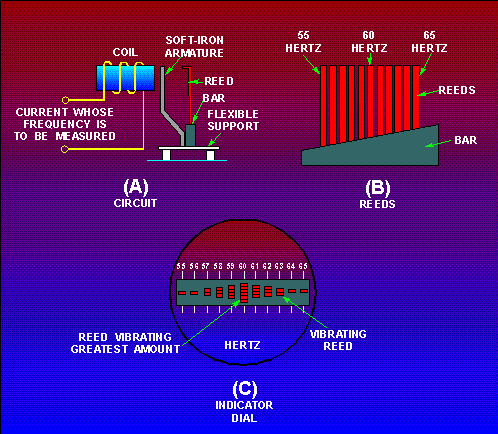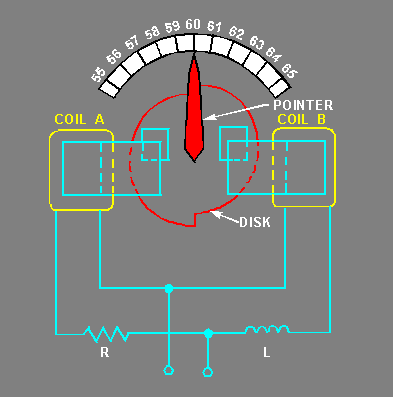Custom SearchFREQUENCY METERS All alternating voltage sources are generated at a set frequency or range of frequencies. A frequency meter provides a means of measuring this frequency. Two common types of frequency meters are the vibrating-reed frequency meter and the moving-disk frequency meter. Vibrating-Reed Frequency Meter The vibrating-reed frequency meter is one of the simplest devices for indicating the frequency of an ac source. Vibrating-reed frequency meters are usually in-circuit meters. They are used on power panels to monitor the frequency of ac. A simplified diagram of one type of vibrating-reed frequency meter is shown in figure 1-47. Figure 1-47. - Simplified disc of a vibrating-reed frequency meter. INDICATOR DIALThe current whose frequency is to be measured flows through the coil and exerts maximum attraction on the soft-iron armature TWICE during each cycle (fig. 1-47(A)). The armature is attached to the bar, which is mounted on a flexible support. Reeds having natural vibration frequencies of 110, 112, 114, and so forth, up to 130 hertz are mounted on the bar (fig. 1-47(B)). The reed having a frequency of 110 hertz is marked 55 hertz; the one having a frequency of 112 hertz is marked 56 hertz; the one having a frequency of 120 hertz is marked 60 hertz; and so forth. When the coil is energized with a current having a frequency between 55 and 65 hertz, all the reeds are vibrated slightly; but, the reed having a natural frequency closest to that of the energizing current (whose frequency is to be measured) vibrates more. The frequency is read from the scale value opposite the reed having the greatest vibration. In some instruments the reeds are the same lengths, but are weighted by different amounts at the top so that they will have different natural rates of vibration. An end view of the reeds is shown in the indicator dial of figure 1-47(C). If the current has a frequency of 60 hertz per second, the reed marked "60" hertz will vibrate the amount, as shown. Moving-Disk Frequency Meter Moving-disk frequency meters are most commonly out-of-circuit meters. They can be used to spot check the frequency of power sources or equipment signals. A moving-disk frequency meter is shown in figure 1-48. One coil tends to turn the disk clockwise, and the other, counterclockwise. Magnetizing coil A is connected in series with a large value of resistance. Coil B is connected in series with a large inductance and the two circuits are supplied in parallel by the source. Figure 1-48. - Simplified diagram of a moving-disk frequency meter.For a given voltage, the current through coil A is practically constant. However, the current through coil B varies with the frequency. At a higher frequency the inductive reactance is greater and the current through coil B is less; the reverse is true at a lower frequency. The disk turns in the direction determined by the stronger coil. A perfectly circular disk would tend to turn continuously. This is not desirable, and so the disk is constructed so that it will turn only a certain amount clockwise or counterclockwise about the center position, which is commonly marked 60 hertz on commercial equipment. To prevent the disk from turning more than the desired amount, the left half of the disk is mounted so that when motion occurs, the same amount of disk area will always be between the poles of coil A. Therefore, the force produced by coil A to rotate the disk is constant for a constant applied voltage. The right half of the disk is offset, as shown in the figure. When the disk rotates clockwise, an increasing area will come between the poles of coil B; when it rotates counterclockwise, a decreasing area will come between the poles of coil B. The greater the area between the poles, the greater will be the disk current and the force tending to turn the disk. If the frequency applied to the frequency meter should decrease, the reactance offered by L would decrease and the field produced by coil B would increase. The field produced by coil A would remain the same. Thus, the force produced by coil B would tend to move the disk and the pointer counterclockwise until the area between the poles was reduced enough to make the two forces equal. The scale is calibrated to indicate the correct frequency. If the frequency is constant and the voltage is changed, the currents in the two coils-and therefore the opposing forces-change by the same amount. Thus, the indication of the instrument is not affected by a change in voltage. Q.64 What are two types of frequency meters?Q.65 What type of meter is shown and what is the value of the quantity being measured for each meter in figure1-49?Integrated Publishing, Inc. - A (SDVOSB) Service Disabled Veteran Owned Small Business